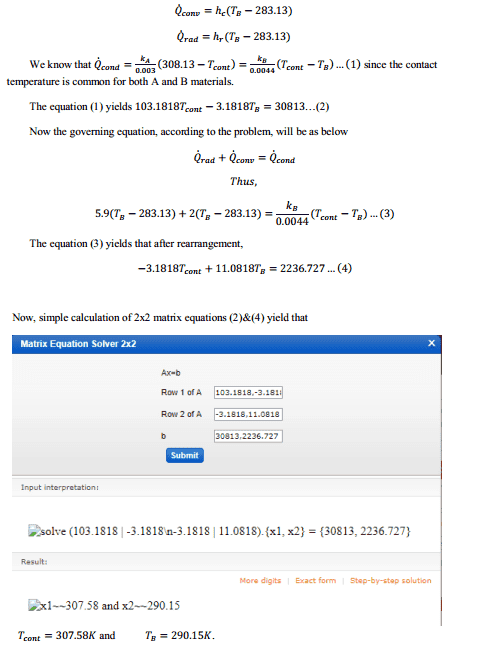# Heat transfer along the joint bar with one side diffusion-convection

sharky444
Homework Statement:
There is given a rectangular bar which is composed of two different materials, X and Y. Cross-section is 1mm width, 1mm of thickness and 3mm length for material X and 4.4mm length for material Y. Assume that all the sides are thermally insulated except left side of the bar where material X has temperature of 308.13K and ambient T=283.13K and right surface of the bar where Y material experiences diffusion and convection . X and Y materials have thermal conductivities of 0.3 W/mK and 0.014W/mK, respectively. Surface emissivity (ε=0.9) and convection ht coefficient h=2W/m^2K. We need to find contact surface temperature.
Ht radiation coefficient is given as h_r = 6W/m^2K.
Relevant Equations:
q=εσ(T_h^4-T_c^4)

There are similar problems with heat conduction only where for example, right side of the bar also has a certain temperature or in-contact with a hot material. However, in this case diffusion and convection occurs on the right side of the bar, more precisely on the Y material. I guess we have to use radiation heat transfer.
I did simulation of this problem and Tcontact was around 304K. Now I need to compare simulation with theoretical value. However, I still could not get the answer

q_cond=kA/L(Tx-Tcontact)

#### Attachments

•heat transfer.png
6.8 KB · Views: 323
Last edited:

Mentor
What is the rate of heat flow out of the bar at the left end?

sharky444
What is the rate of heat flow out of the bar at the left end?
Sir, it is not given in the problem. Just the temperature Tx=308.13K...

Mentor
Sir, it is not given in the problem. Just the temperature Tx=308.13K...
So you are saying that you don't know how to use the equation q=εσ(T_h^4-T_c^4) to calculate the heat flux at the left end, given T_h = 308.13 K and T_c = 283.13 K?

•sharky444
sharky444
So you are saying that you don't know how to use the equation q=εσ(T_h^4-T_c^4) to calculate the heat flux at the left end, given T_h = 308.13 K and T_c = 283.13 K?
Thank you. I have figured out it. I totally forgot about ambient temperature

Mentor
Thank you. I have figured out it. I totally forgot about ambient temperature
So you have the full solution now?

•SujeeS
sharky444
So you have the full solution now?
Sir, to be honest, not yet. I have just tried to do simulation (comsol multiphysics) again and it appeared to be T_contact=306K.
This is my attempt (since I am not that good in heat transfer, main equation might be wrong that is where I am stuck).
-Qrad-Qconv=k_x/L(308.13-T_contact) and after rigorous calculation I got around T_contact=286K.
Could you please kindly point where my mistake is?

Mentor
Sir, to be honest, not yet. I have just tried to do simulation (comsol multiphysics) again and it appeared to be T_contact=306K.
This is my attempt (since I am not that good in heat transfer, main equation might be wrong that is where I am stuck).
-Qrad-Qconv=k_x/L(308.13-T_contact) and after rigorous calculation I got around T_contact=286K.
Could you please kindly point where my mistake is?
The heat is flowing along the bar from right to left. If Qrad is the heat flux at the left boundary due to radiation, this is also the heat flux by conduction between the contact point and the left boundary. Therefore, $$Q_{cond}=k_x\frac{(T_{contact}-308.13)}{0.003}=Q_{rad}$$

sharky444
The heat is flowing along the bar from right to left. If Qrad is the heat flux at the left boundary due to radiation, this is also the heat flux by conduction between the contact point and the left boundary. Therefore, $$Q_{cond}=k_x\frac{(T_{contact}-308.13)}{0.003}=Q_{rad}$$
I have solved it and confirmed with the simulation. The simulation values are T_cont=307.59K (recalculated) and Ty=290.72K which coincide with analytical solution. Note that the rate of Q_rad is determined by the heat radiation coefficient. (x and y are the same materials as A and B in the solution, respectively)Mentor
I have solved it and confirmed with the simulation. The simulation values are T_cont=307.59K (recalculated) and Ty=290.72K which coincide with analytical solution. Note that the rate of Q_rad is determined by the heat radiation coefficient. (x and y are the same materials as A and B in the solution, respectively)
View attachment 243973
None of this makes any sense to me. Maybe the problem statement is ambiguous and subject to interpretation. I've had a huge amount of practical experience with heat transfer, and there is no way I could get the answer wrong if they were precise with what they were trying to specify.